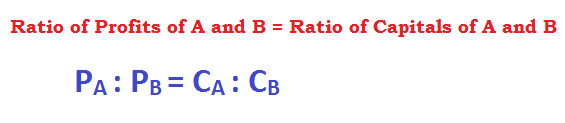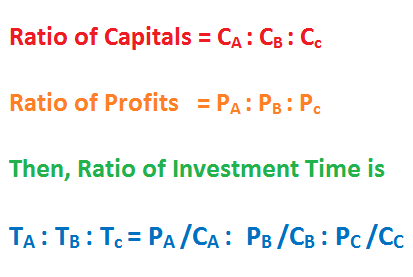## Partnership

People enter into partnership to accumulate money to kick start a business.

The money invested by each is called Capital.

Once the business starts to generate income, each partner will ask for his own share of profit.

The question is how much will each receive as his due share?

Two criteria are applied during division of profit among the partners:

• Profit is divided in the ratio of capitals invested by each, if each partner invests for a same duration.Example 1:

John and Mike invest \$10000 and \$12000 for one year each to start some small business. In an income of \$110 at the end of the year, what is the share of each?

Solution:

Both invest for a same time period. Therefore, profit will be shared in ratio of their capitals.

Ratio of capitals = 10000: 12000 = 5: 6

John’s share = [5/ (5 + 6)] × 110 = 5/11(110) = 50, and

Mike’s share = 110 – 50 = 60, Or

= [6/ (5 + 6)] × 110 = 6/11(110) = 60

• Profit is divided in the ratio of product of capital and duration of time that each partner invests for.Sometimes, each partner invests money for varying time periods. When capitals and time period of investments are different, then profit will have to be shared in proportion to both capital and time.

In this case, profit is shared in the ratio of product of capital and time.Example 1:

Jim and Nancy invest \$2000 and \$2500 to buy some stock. They agree to divide the profit only at the end of the year. Nancy withdrew her money after 8 months.How much did each receive if a profit of \$550 was made at the end of the year?

Solution:

Each has invested different money and for different time period.

Therefore, profit will be shared in ratio of product of capital and time.

Ratio of profit of Jim and Nancy =

2000 × 12: 2500 × 8 = 24000: 20000 = 24: 20 = 6: 5

Jim’s profit = [6/ (6 + 5)] × 550 = 6/11(550) = 300 and

Nancy’s profit = [5/ (5 + 6)] × 550 = 5/11(110) = 250

Or better, Nancy’s profit = 550 – 300 = 250

So, in the profit of \$550, Jim receives \$300 and Nancy, \$250

Very Important Formula:

Let ratio of capitals be CA : CB : Cc

Let the ratio of profits be PA : PB : Pc

Then ratio of time periods of investment is:Example 3:

Three persons invested \$200, \$250 and \$300 to make some income. The profit was shared in the ratio 4: 5: 6 at the end of the year. How long did each invest themoney for?

Solution:

Ratio of capitals = 200: 250: 300 = 20: 25: 30 = 4: 5: 6

Ratio of duration of investment = 4/4: 5/5: 6/6 = 1: 1: 1

Example 4:

John put \$500 to start off a website. Mike joined him with \$800 after some time. At the end of the year, they shared the profit in the ratio 3: 4. When did Mike join?

Solution:

Let Mike invest for‘t’ months

(Profit of John)/ (Profit of Mike) = (Capital × Time) of John/ (Capital × Time) of Mike.

3/4 = (500 × 12)/ (800 × t), i.e.

3/4 = (5 × 12)/ (8 × t), so t = 10

So, Mike invested for 10 months.

Mike joined two months after John started.

Example 5:

Three persons A, B and C agree to work for a contract of \$15000. A completes half of the work, while B completes half of the remaining and C the rest of the work. How much income will C receive as his share?

Solution:

A completes half of the work. So, another half is left.

B and C each do half of the

C completes = ½ (Half left after A finishes half of the work) = ½ × (1/2) = ¼